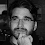## Friday, January 2, 2015

### File.ReadAllText with an Offset in .NETWhat do you do when you need to read text from a file in .NET, but you want to start from an offset? This is a slightly niche scenario, but it does happen. Below is a solution to that problem.

To summarize the implementation, you open a file stream, seek to your offset, and then read in the bytes from there. While loading the result I read small chunks into a buffer, decoded them, and then added the decoded string to a string buffer for storage. Please note that if you are using a multibyte encoding then this helper will only work if you use the correct offset.

### Helper Code

`public class FileHelper`
`{`
`    private const int BufferSize = 1024;`
` `
`    public static string ReadAllTextFromOffset(`
`        string path, `
`        Encoding encoding, `
`        int offset, `
`        out int totalLength)`
`    {`
`        using (var fs = new FileStream(path, FileMode.Open))`
`        {`
`            totalLength = offset;`
` `
`            if (offset > 0)`
`            {`
`                fs.Seek(offset, SeekOrigin.Begin);`
`            }`
` `
`            var sb = new StringBuilder();`
`            var buffer = new byte[BufferSize];`
`            int readCount;`
` `
`            do`
`            {`
`                readCount = fs.Read(buffer, 0, buffer.Length);`
`                totalLength += readCount;`
` `
`                var subString = encoding.GetString(buffer, 0, readCount);`
`                sb.Append(subString);`
`            }`
`            while (readCount == buffer.Length);`
` `
`            return sb.ToString();`
`        }`
`    }`
`}`

### Test Code

`public class FileHelperTests`
`{`
`    private const string Path = "C:/Code/";`
` `
`    [Fact]`
`    public void ReadAllTextWithOffset()`
`    {`
`        const string path = Path + "ReadAllTextWithOffset.txt";`
`        var encd = Encoding.Default;`
` `
`        try`
`        {`
`            File.WriteAllText(path, "Hello World Goodnight Moon", encd);`
` `
`            int al;`
`            var a = FileHelper.ReadAllTextFromOffset(path, encd, 0, out al);`
` `
`            int bl;`
`            var b = FileHelper.ReadAllTextFromOffset(path, encd, 2, out bl);`
`            Assert.True(a.EndsWith(b));`
`            Assert.Equal(a.Length - 2, b.Length);`
` `
`            int cl;`
`            var c = FileHelper.ReadAllTextFromOffset(path, encd, 12, out cl);`
`            Assert.True(a.EndsWith(c));`
`            Assert.Equal(a.Length - 12, c.Length);`
`        }`
`        finally`
`        {`
`            File.Delete(path);`
`        }`
`    }`
` `
`    [Fact]`
`    public void ReadAllTextWithOffsetTooFar()`
`    {`
`        const string path = Path + "ReadAllTextWithOffsetTooFar.txt";`
`        var encd = Encoding.Default;`
` `
`        try`
`        {`
`            File.WriteAllText(path, "Hello World Goodnight Moon", encd);`
` `
`            int l;`
`            var s = FileHelper.ReadAllTextFromOffset(path, encd, 128, out l);`
`            Assert.Equal(string.Empty, s);`
`            Assert.Equal(128, l);`
`        }`
`        finally`
`        {`
`            File.Delete(path);`
`        }`
`    }`
` `
`    [Fact]`
`    public void ReadAllTextWithOffsetWithUpdates()`
`    {`
`        const string path = Path + "ReadAllTextWithOffsetWithUpdates.txt";`
`        var encd = Encoding.Default;`
` `
`        try`
`        {`
`            var a = "Hello World" + Environment.NewLine;`
`            File.AppendAllText(path, a, encd);`
` `
`            int al;`
`            var at = FileHelper.ReadAllTextFromOffset(path, encd, 0, out al);`
`            Assert.Equal(a, at);`
` `
`            var b = "Goodnight Moon" + Environment.NewLine;`
`            File.AppendAllText(path, b, encd);`
` `
`            int bl;`
`            var bt = FileHelper.ReadAllTextFromOffset(path, encd, al, out bl);`
`            Assert.Equal(b, bt);`
`        }`
`        finally`
`        {`
`            File.Delete(path);`
`        }`
`    }`
`}`

Enjoy,
Tom

1.1.